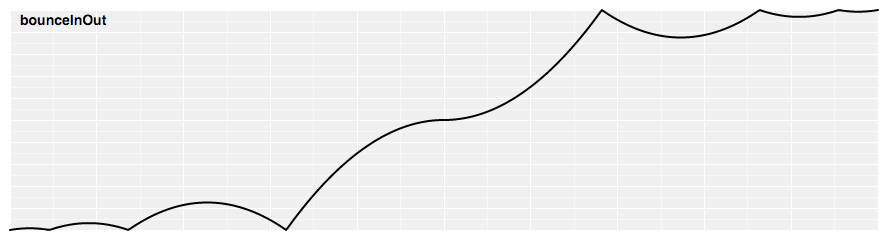/D3.js 5

# d3-ease

Easing is a method of distorting time to control apparent motion in animation. It is most commonly used for slow-in, slow-out. By easing time, animated transitions are smoother and exhibit more plausible motion.

The easing types in this module implement the ease method, which takes a normalized time t and returns the corresponding “eased” time . Both the normalized time and the eased time are typically in the range [0,1], where 0 represents the start of the animation and 1 represents the end; some easing types, such as elastic, may return eased times slightly outside this range. A good easing type should return 0 if t = 0 and 1 if t = 1. See the easing explorer for a visual demonstration.

These easing types are largely based on work by Robert Penner.

## Installing

If you use NPM, `npm install d3-ease`. Otherwise, download the latest release. You can also load directly from d3js.org, either as a standalone library or as part of D3 4.0. AMD, CommonJS, and vanilla environments are supported. In vanilla, a `d3` global is exported:

```<script src="https://d3js.org/d3-ease.v1.min.js"></script>
<script>

var ease = d3.easeCubic;

</script>```

## API Reference

###### ease(t)

Given the specified normalized time t, typically in the range [0,1], returns the “eased” time , also typically in [0,1]. 0 represents the start of the animation and 1 represents the end. A good implementation returns 0 if t = 0 and 1 if t = 1. See the easing explorer for a visual demonstration. For example, to apply cubic easing:

`var te = d3.easeCubic(t);`

Similarly, to apply custom elastic easing:

```// Before the animation starts, create your easing function.
var customElastic = d3.easeElastic.period(0.4);

// During the animation, apply the easing function.
var te = customElastic(t);```
###### d3.easeLinear(t) Source

Linear easing; the identity function; linear(t) returns t.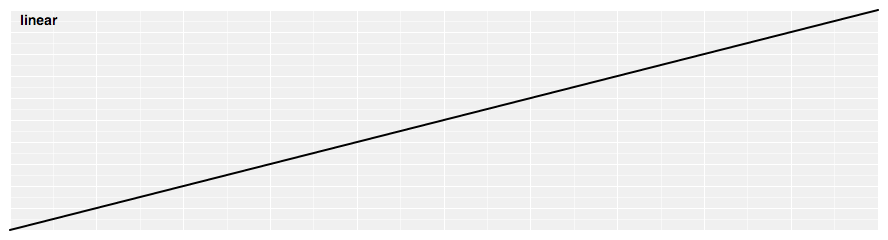###### d3.easePolyIn(t) Source

Polynomial easing; raises t to the specified exponent. If the exponent is not specified, it defaults to 3, equivalent to cubicIn.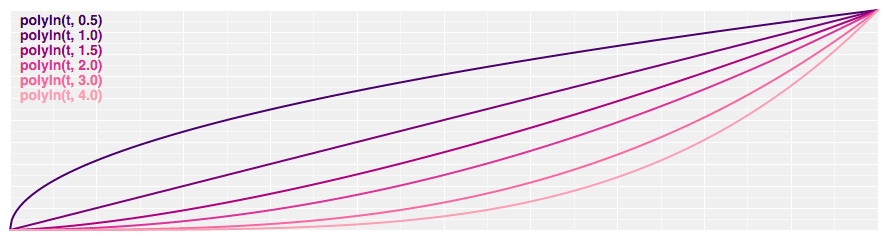###### d3.easePolyOut(t) Source

Reverse polynomial easing; equivalent to 1 - polyIn(1 - t). If the exponent is not specified, it defaults to 3, equivalent to cubicOut.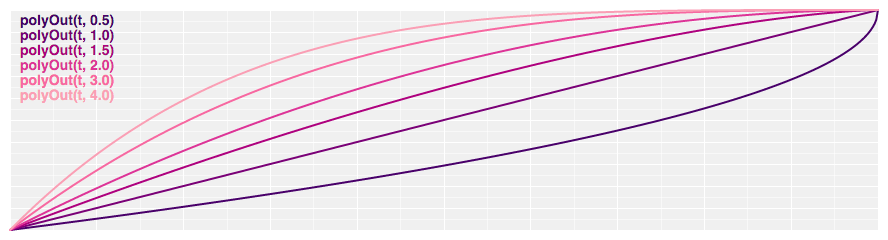###### d3.easePoly(t) Source d3.easePolyInOut(t) Source

Symmetric polynomial easing; scales polyIn for t in [0, 0.5] and polyOut for t in [0.5, 1]. If the exponent is not specified, it defaults to 3, equivalent to cubic.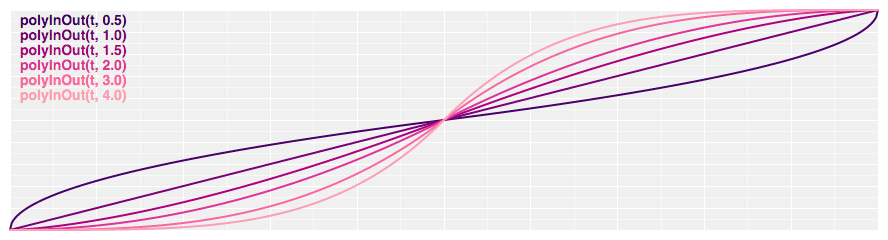###### poly.exponent(e) Source

Returns a new polynomial easing with the specified exponent e. For example, to create equivalents of linear, quad, and cubic:

```var linear = d3.easePoly.exponent(1),
cubic = d3.easePoly.exponent(3);```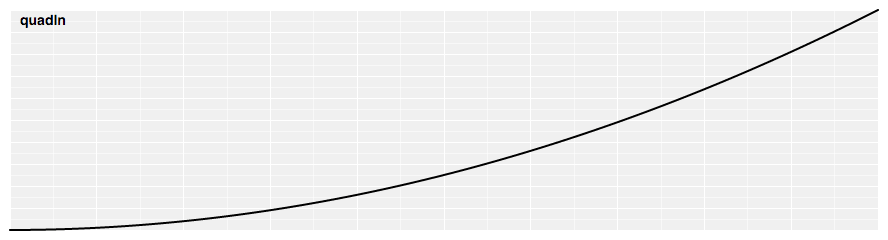Reverse quadratic easing; equivalent to 1 - quadIn(1 - t). Also equivalent to polyOut.exponent(2).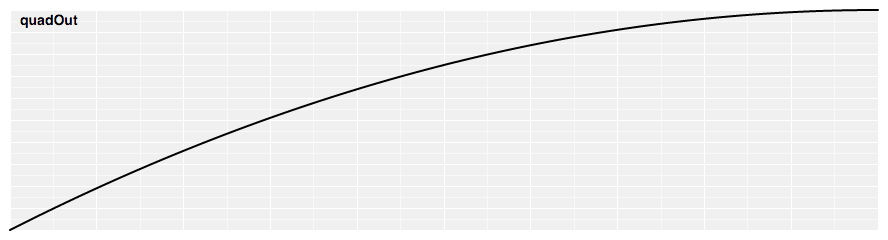Symmetric quadratic easing; scales quadIn for t in [0, 0.5] and quadOut for t in [0.5, 1]. Also equivalent to poly.exponent(2).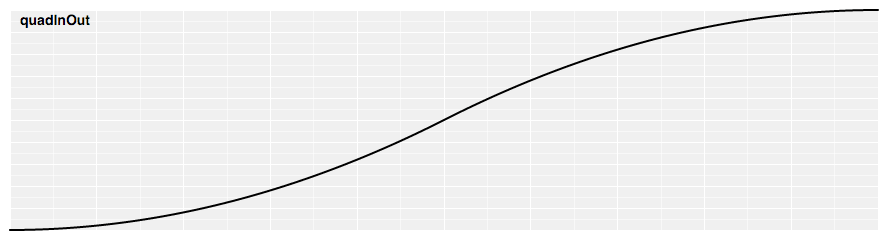###### d3.easeCubicIn(t) Source

Cubic easing; equivalent to polyIn.exponent(3).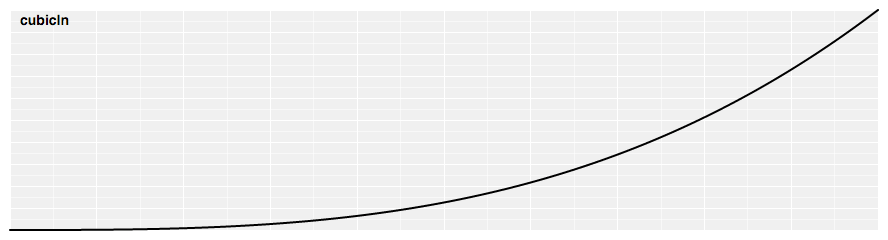###### d3.easeCubicOut(t) Source

Reverse cubic easing; equivalent to 1 - cubicIn(1 - t). Also equivalent to polyOut.exponent(3).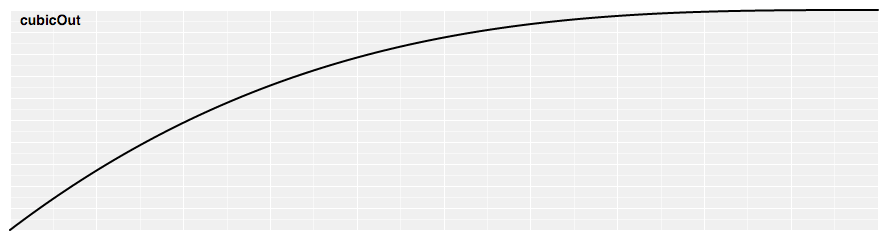###### d3.easeCubic(t) Source d3.easeCubicInOut(t) Source

Symmetric cubic easing; scales cubicIn for t in [0, 0.5] and cubicOut for t in [0.5, 1]. Also equivalent to poly.exponent(3).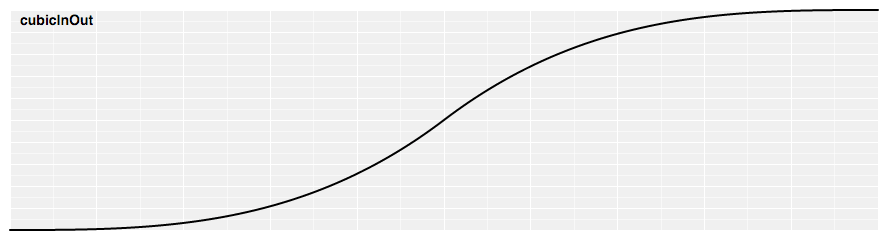###### d3.easeSinIn(t) Source

Sinusoidal easing; returns sin(t).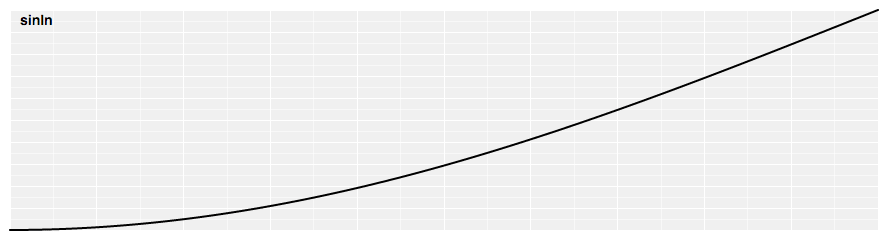###### d3.easeSinOut(t) Source

Reverse sinusoidal easing; equivalent to 1 - sinIn(1 - t).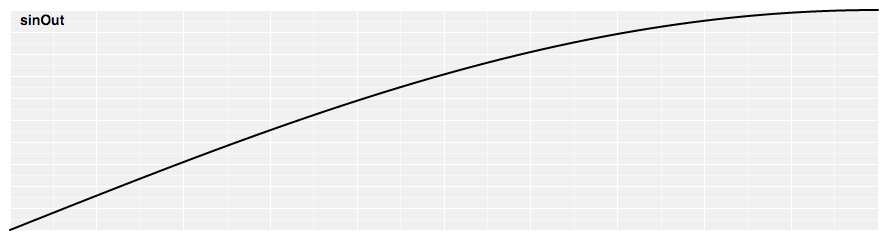###### d3.easeSin(t) Source d3.easeSinInOut(t) Source

Symmetric sinusoidal easing; scales sinIn for t in [0, 0.5] and sinOut for t in [0.5, 1].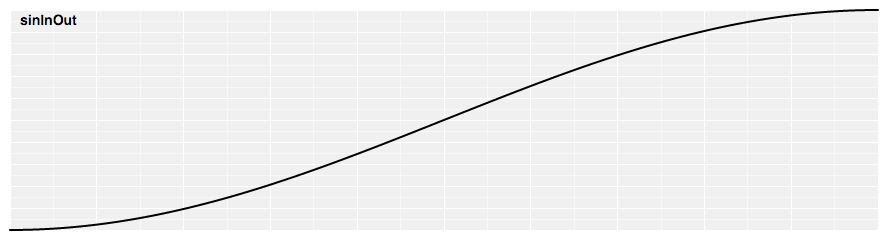###### d3.easeExpIn(t) Source

Exponential easing; raises 2 to the exponent 10 * (t - 1).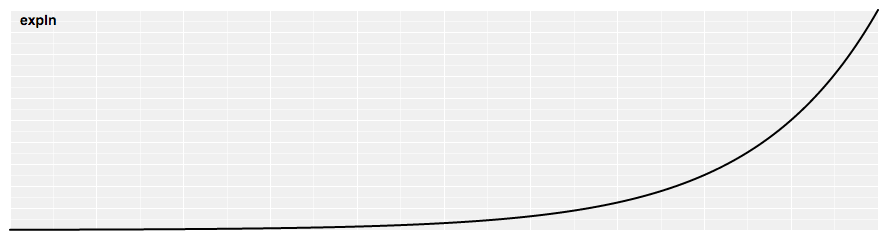###### d3.easeExpOut(t) Source

Reverse exponential easing; equivalent to 1 - expIn(1 - t).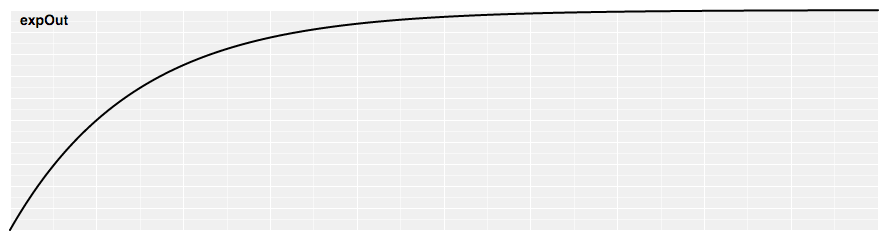###### d3.easeExp(t) Source d3.easeExpInOut(t) Source

Symmetric exponential easing; scales expIn for t in [0, 0.5] and expOut for t in [0.5, 1].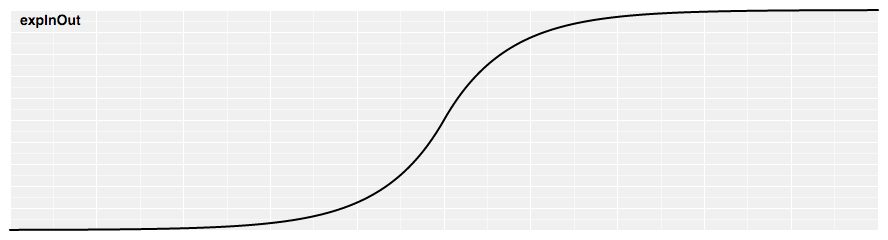###### d3.easeCircleIn(t) Source

Circular easing.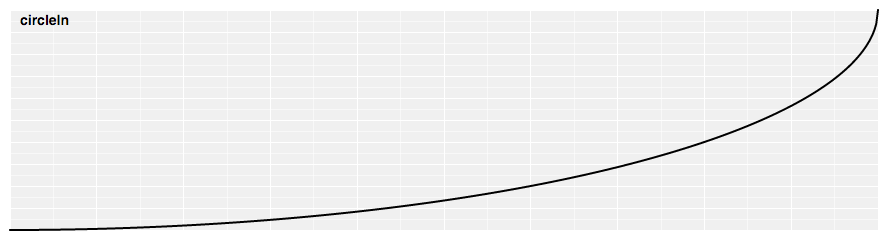###### d3.easeCircleOut(t) Source

Reverse circular easing; equivalent to 1 - circleIn(1 - t).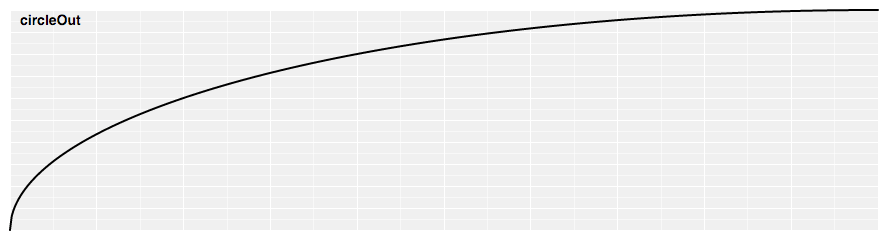###### d3.easeCircle(t) Source d3.easeCircleInOut(t) Source

Symmetric circular easing; scales circleIn for t in [0, 0.5] and circleOut for t in [0.5, 1].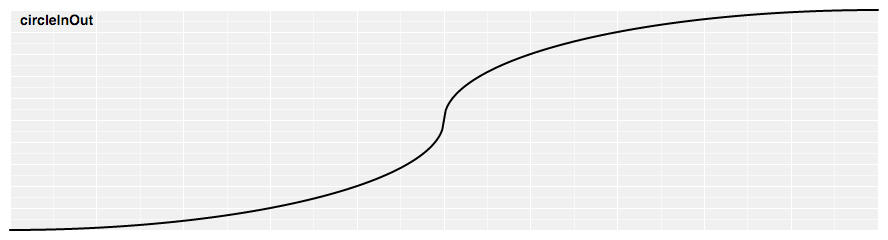###### d3.easeElasticIn(t) Source

Elastic easing, like a rubber band. The amplitude and period of the oscillation are configurable; if not specified, they default to 1 and 0.3, respectively.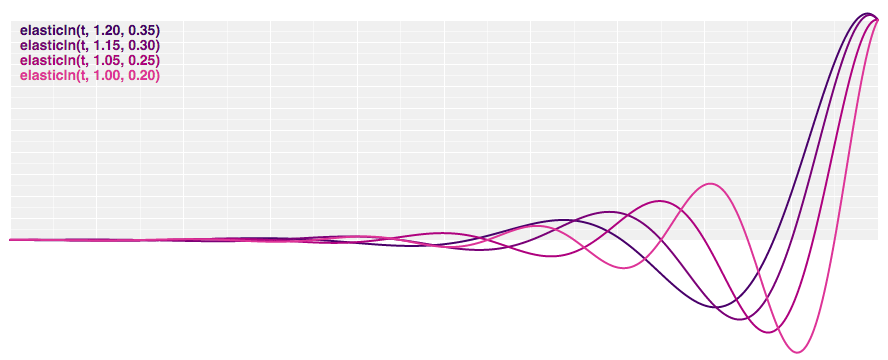###### d3.easeElastic(t) Source d3.easeElasticOut(t) Source

Reverse elastic easing; equivalent to 1 - elasticIn(1 - t).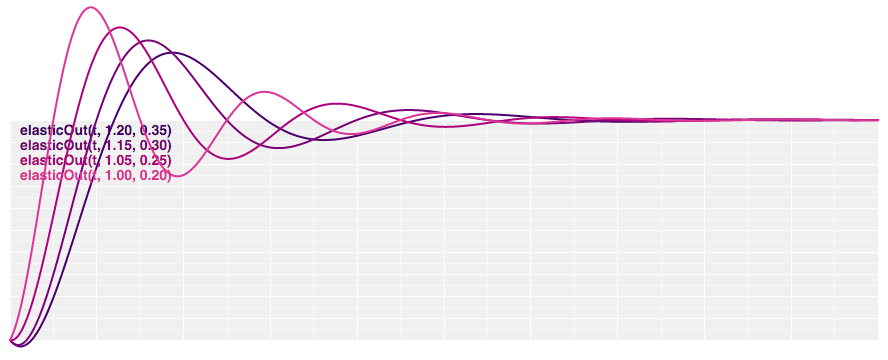###### d3.easeElasticInOut(t) Source

Symmetric elastic easing; scales elasticIn for t in [0, 0.5] and elasticOut for t in [0.5, 1].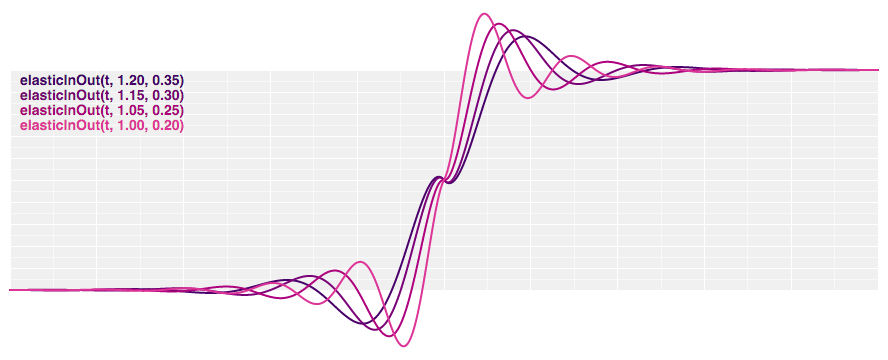###### elastic.amplitude(a) Source

Returns a new elastic easing with the specified amplitude a.

###### elastic.period(p) Source

Returns a new elastic easing with the specified period p.

###### d3.easeBackIn(t) Source

Anticipatory easing, like a dancer bending his knees before jumping off the floor. The degree of overshoot is configurable; it not specified, it defaults to 1.70158.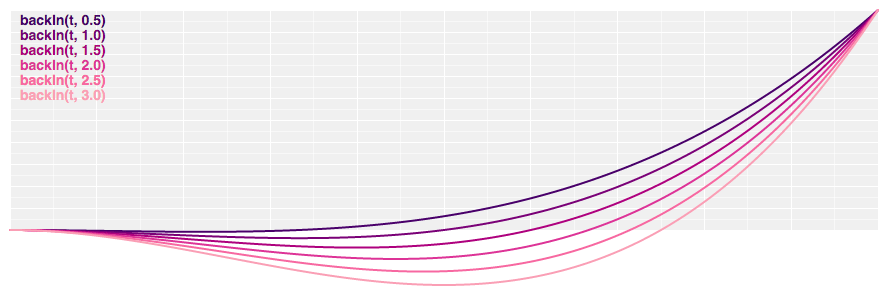###### d3.easeBackOut(t) Source

Reverse anticipatory easing; equivalent to 1 - backIn(1 - t).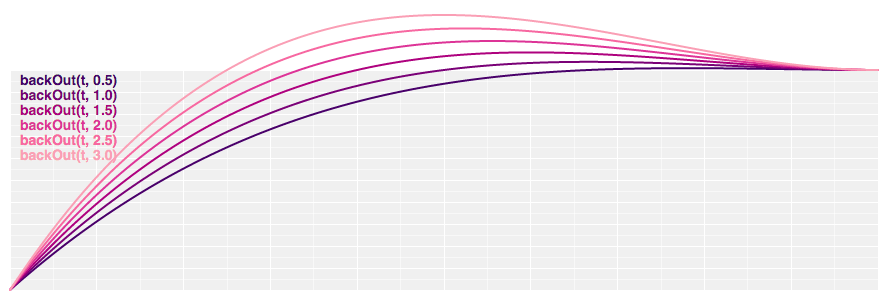###### d3.easeBack(t) Source d3.easeBackInOut(t) Source

Symmetric anticipatory easing; scales backIn for t in [0, 0.5] and backOut for t in [0.5, 1].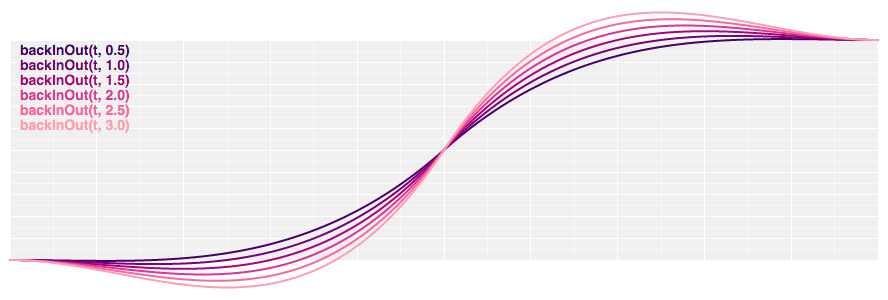###### back.overshoot(s) Source

Returns a new back easing with the specified overshoot s.

###### d3.easeBounceIn(t) Source

Bounce easing, like a rubber ball.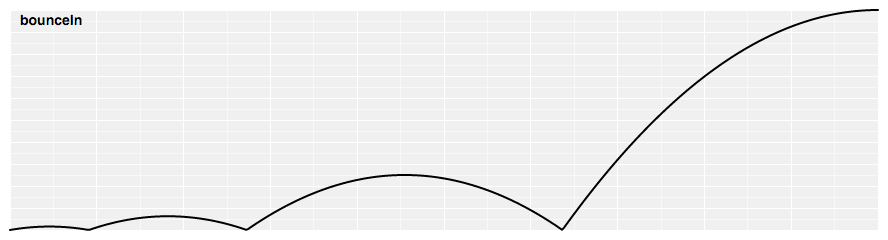###### d3.easeBounce(t) Source d3.easeBounceOut(t) Source

Reverse bounce easing; equivalent to 1 - bounceIn(1 - t).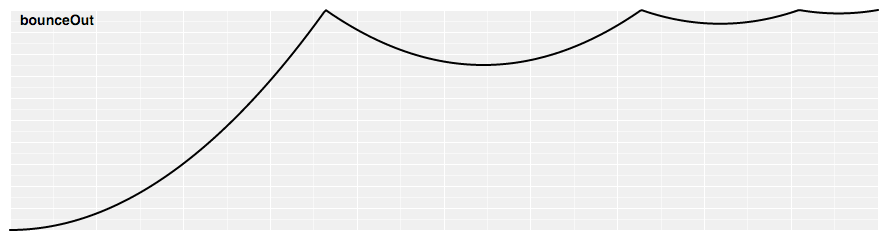###### d3.easeBounceInOut(t) Source

Symmetric bounce easing; scales bounceIn for t in [0, 0.5] and bounceOut for t in [0.5, 1].